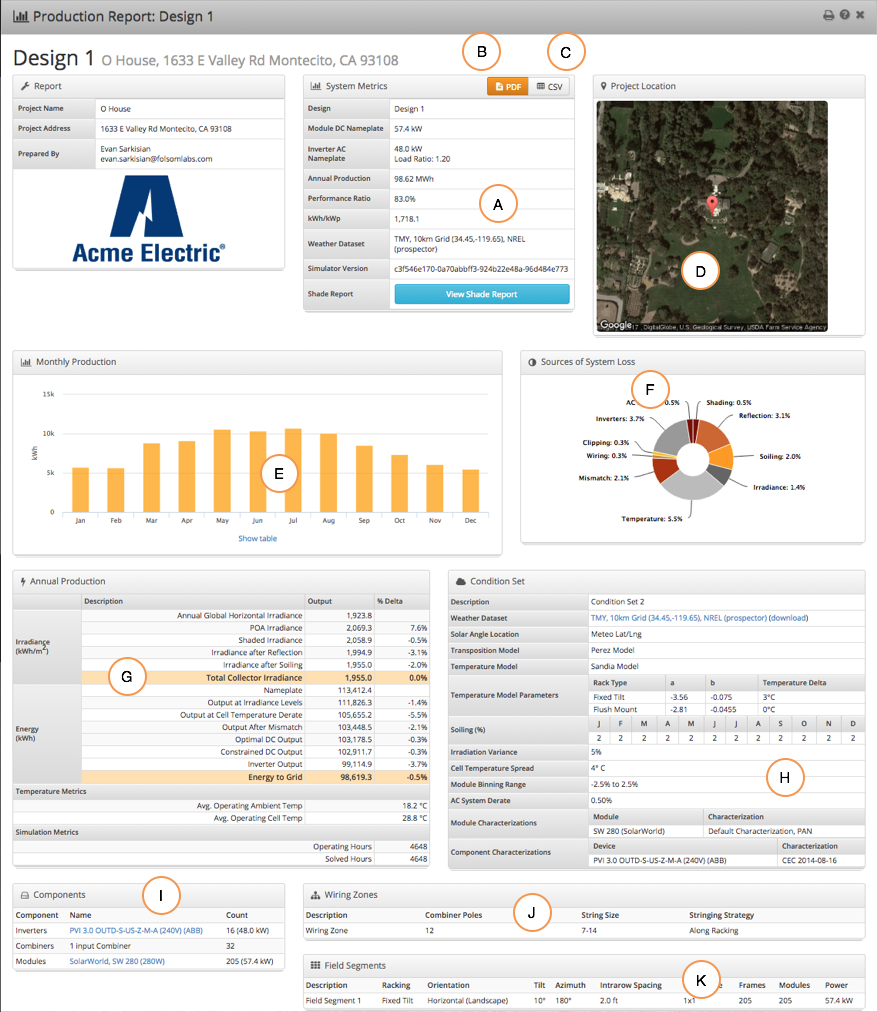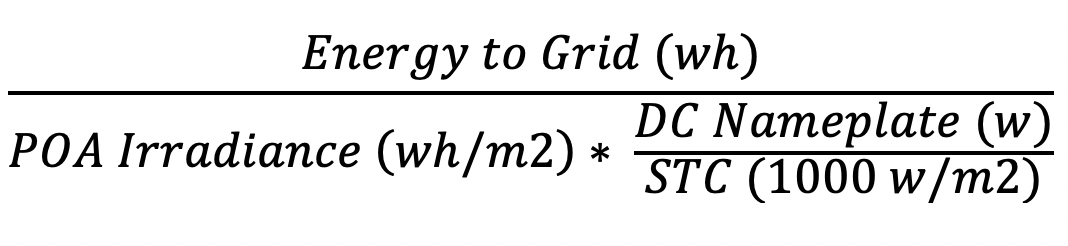# Production Report Summary

### Overview of Production Report Layout

A detailed Report is available for each completed Simulation:

A. Summary metrics, including energy production, performance ratio, and kWh/kWp
B. Click the orange PDF button to download a printable PDF report
C. Hourly results can be downloaded in CSV format
D. Project location is shown overhead
E. Monthly production values
F. Chart shows loss factors graphically
G. Detailed loss tree shows the total losses at each step of the irradiance and energy calculations.
H. Records of condition set assumptions
I. Bill of materials the Design, including module quantity, wire quantity, and inverter quantities
J. Detail on electrical design assumptions
K. Detail on mechanical layout and module orientation### Glossary of Terms

Summary Metrics:

 Production The total energy generated during the simulation, in MWh or GWh Performance Ratio Performance Ratio (PR) shows the percentage of total potential energy for the array that is converted to AC energy.  Mathematically, the PR is defined in the following formula:In the report above we find the following values: Energy to Grid: 98,619.3 kWh DC Nameplate: 57.5 kW POA Irradiance: 2,069.3 kWh/m2 We can calculate the performance ratio as 98,619.3 / (2069.3 * 57.5) = 82.9%  Note: rounding in POA Irradiance and DC nameplate can cause slight rounding errors in the calculation. To get the full POA irradiance value, you would need to use the hourly CSV export. To get full DC nameplate multiply module count by module wattage.  Shading will reduce the PR, since it reduces the AC power, but does not reduce the POA irradiance.  However, differences in orientation angle will have very little effect on PR, since the POA irradiance will change with the module orientation.   * STC stands for Standard Test Conditions. These are measured under lab conditions of 1000W per sq meter of irradiance and 25 degree C. kWh/kWp The total simulation energy generation divided by the system DC nameplate power.

Production Metrics

 Annual Global Horizontal Irradiance The total irradiance that will fall on a flat plane at the location of the array.  This is aggregated directly from the weather file. POA Irradiance The total irradiance in the plane of the modules, accounting for tilt and azimuth angles.  This is averaged across all modules in the array. Shaded Irradiance The total irradiance accounting for all shading (from horizon, row-to-row, and obstruction) Irradiance after Reflection The total irradiance after accounting for reflection off the surface of the module (i.e. IAM reflection). Irradiance after Soiling Irradiance after module soiling is accounted for.  Note that soiling assumptions are made in the Condition Set. Total Collector Irradiance The total annual irradiance available to the modules in the array.  This is averaged across all modules. Nameplate The maximum potential power of the array, defined as the total collector irradiance multiplied by the system nameplate power. Output at Irradiance Levels The total energy output by the modules, after accounting for low-light effects and module IV curve distortions. More details on Output at Irradiance available here. Output at Cell Temperature The total output of the modules, factoring in the temperature effects on the IV curves.  This is the sum of the modules at their maximum power points. Output After Mismatch The total energy output of the modules, factoring in all system constraints (e.g. series & parallel mismatch, voltage drop, etc.). Optimizer Output If DC optimizers are present, this shows the total output of the optimizers, factoring in their efficiency curves and principles of operation. Optimal DC Output The total energy available energy of the DC system, accounting for all wire resistive losses. Constrained DC Output The total DC energy after accounting for inverter voltage and power limits. More details about clipping and Constrained DC Output available here Inverter Output The total AC energy output from the inverters, taking into account inverter performance losses. Energy to Grid The total AC energy output after accounting for AC wire losses to the point of interconnection